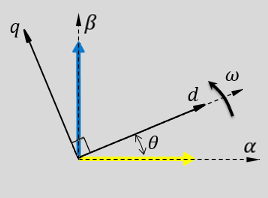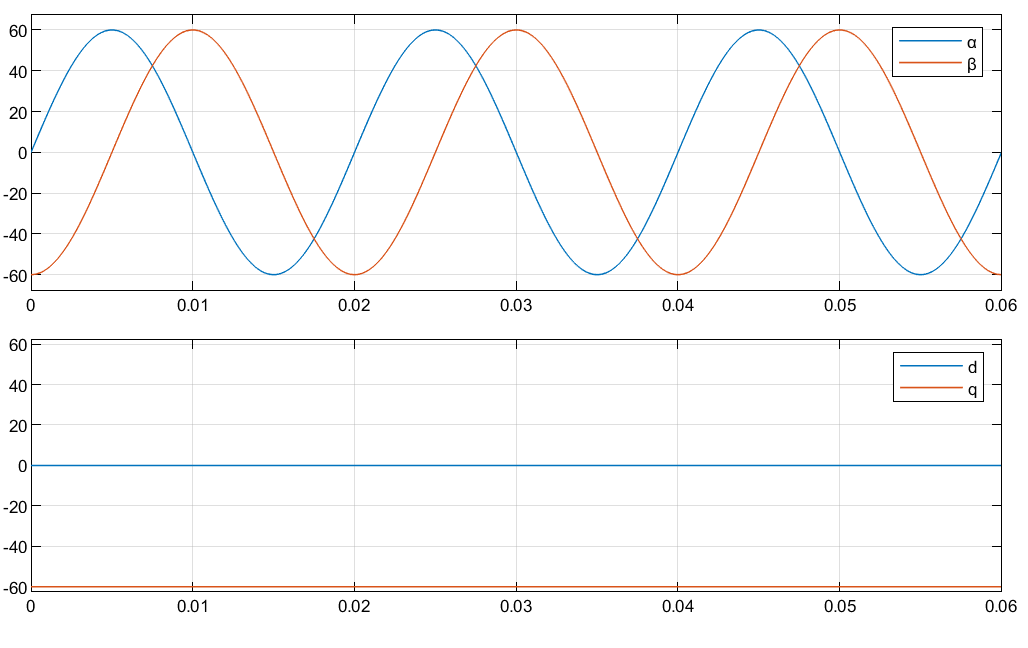# Park Transform

Implement αβ to dq transformation

• Library:
• Motor Control Blockset / Controls / Math Transforms

•## Description

The Park Transform block computes the Park transformation of two-phase orthogonal components in a stationary αβ reference frame.

The block accepts the following inputs:

• α-β axes components in the stationary reference frame.

• Sine and cosine values of the corresponding angles of transformation.

It outputs orthogonal direct and quadrature axis components in the rotating dq reference frame. You can configure the block to align either the d- or the q-axis with the α-axis at time t = 0.

The figures show the α-β axes components in an αβ reference frame and a rotating dq reference frame for when:

• The d-axis aligns with the α-axis.• The q-axis aligns with the α-axis.In both cases, the angle θ = ωt, where:

• θ is the angle between the α- and d-axes for the d-axis alignment or the angle between the α- and q-axes for the q-axis alignment. It indicates the angular position of the rotating dq reference frame with respect to the α-axis.

• ω is the rotational speed of the d-q reference frame.

• t is the time, in seconds, from the initial alignment.

The figures show the time-response of the individual components of the αβ and dq reference frames when:

• The d-axis aligns with the α-axis.• The q-axis aligns with the α-axis.### Equations

The following equations describe how the block implements Park transformation.

• When the d-axis aligns with the α-axis.

• When the q-axis aligns with the α-axis.

where:

• ${f}_{\alpha }$ and ${f}_{\beta }$ are the two-phase orthogonal components in the stationary αβ reference frame.

• ${f}_{d}$ and ${f}_{q}$ are the direct and quadrature axis orthogonal components in the rotating dq reference frame.

## Ports

### Input

expand all

Alpha-axis component, α, in the stationary αβ reference frame.

Data Types: `single` | `double` | `fixed point`

Beta-axis component, β, in the stationary αβ reference frame.

Data Types: `single` | `double` | `fixed point`

Sine value of the angle of transformation, θe. θe is the angle between the rotating reference frame and the α-axis.

Data Types: `single` | `double` | `fixed point`

Cosine value of the angle of transformation, θe. θe is the angle between the rotating reference frame and the α-axis.

Data Types: `single` | `double` | `fixed point`

### Output

expand all

Direct axis component, d, in the rotating dq reference frame.

Data Types: `single` | `double` | `fixed point`

Quadrature axis component, q, in the rotating dq reference frame.

Data Types: `single` | `double` | `fixed point`

## Parameters

expand all

Align either the d- or q-axis of the rotating reference frame to the α-axis of the stationary reference frame.